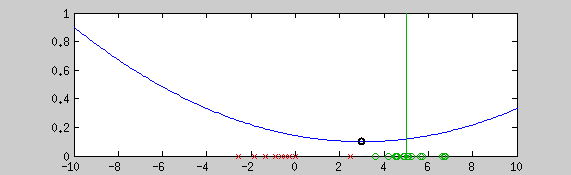# Graduate non convexity approach for robust fittingThis example shows the estimation of the mean with the graduate non convexity approach using a newton optimization at each step for locally minimizing the energy. Green points are a realization of a normal distribution (vertical line shows the mean), red crosses are outliers. The blue line is the normalized …